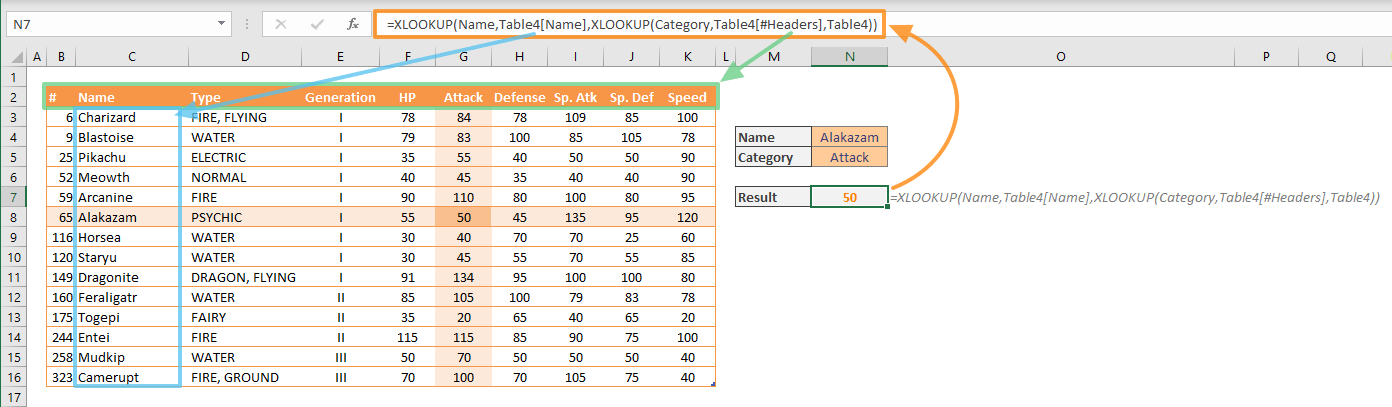The XLOOKUP function is a Lookup & Reference function that can find values in a table or a range by row. Similar to the better-known VLOOKUP function, you can use this function to do things like looking up the name or price of a product by its code number. In this guide, we’re going to show you how to use the XLOOKUP function and also go over some tips and error handling methods.

## Supported versions

• Office 365 Subscribers only

## Syntax of the XLOOKUP Function

XLOOKUP(lookup_value, lookup_array, return_array, [if_not_found], [match_mode], [search_mode])

## Arguments

 lookup_value The value you are looking for lookup_array Where you are looking; the array or range where you want to look up the value return_array The array or range where you want to get return value [if_not_found] Optional. Text to be displayed if there is no match. #N/A will be returned if omitted. [match_mode] Optional. A predefined number specifying the match type:  0: Exact match. If none found, returns #N/A. Default option. -1: Exact match. If none found, returns the next smaller item.  1: Exact match. If none found, returns the next larger item.  2: A wildcard match where *, ?, and ~ characters have a specific purpose. [search_mode] Optional. A predefined number specifying the search mode:  1: Perform a search starting with the first item. This is the default option. -1: Perform a reverse search starting with the last item.  2: Perform a binary search that relies on lookup_array being sorted in ascending order. If not sorted, invalid results will be returned. -2: Perform a binary search that relies on lookup_array being sorted in descending order. If not sorted, invalid results will be returned.

## Examples of XLOOKUP Function

### Basic lookup

XLOOKUP needs at least 3 arguments to work: the value you are looking for, an array or a range that may include your search value, and an array or a range for return values.

When only these 3 arguments are supplied, the XLOOKUP tries to find the exact value, starting from the top of the list. In our example, we are searching in the Name column of the table and want to get the corresponding data in Id, Generation and Attack columns.

To get Generation: =XLOOKUP(Name,Table1[Name],Table1[Generation])You can use the [if_not_found] argument to show a specific message to the user if XLOOKUP could not find the search value. This argument saves you from wrapping the formula inside IFERROR or other error handling functions.

The following function returns the specified message when no matches are found.

=XLOOKUP(Name,Table2[Name],Table2[Attack],"Pokémon is not existed in the list")### Return array

The XLOOKUP function can also return multiple values as an array. To do this, you need to supply a 2-dimensional array or range. The function returns the entire row or column based on the search direction. With Excel’s dynamic arrays feature, all values in the return array will be populated (spilled) into multiple cells.

The following example resembles with the first (Basic Lookup) example, with one difference: The return array has multiple columns, instead of one. Thus, a single formula returns all values in the corresponding row.

=XLOOKUP(Name,Table15[Name],Table15[[HP]:[Speed]])### Two-way lookup

You can use the XLOOKUP to find an intersection of two values. The approach is like in the INDEX-MATCH combination. However, this time you need to use two nested XLOOKUP formulas.

The logic behind this formula is to supply an array for the return array of the wrapper function. In the following example, the inner XLOOKUP searches Category in the headers of the table and returns the values in matched column of the table. On the other hand, the outer XLOOKUP searches Name and looks the return value from the inner XLOOKUP’s return array.### Approximate match

Approximate searches for approximate results if the exact match is not found. Tax bracket search is a good example for this option. You can search your income value and find which tax bracket you are in.

With this, you can also specify a direction for the approximation with the [match_type] value.

Cheat Sheet:

• -1: Exact match. If none found, return the next smaller item.
• 1: Exact match. If none found, return the next larger item.

In our example, we are searching for 64 in the Defense column. The nearest values are 55 and 65. Here are the formulas to get both values.### Wildcard support

XLOOKUP function can also be used for wildcard character support. Wildcard is a term for a character that can represent one or more “unknown” characters. If you are unfamiliar with the wildcard concept in Excel, please visit How to use Wildcard criteria in Excel formulas.

Cheat Sheet:

• Asterisk (*)             Any value (zero or greater)
• Question mark (?) Any single character
• Tilde (~)                  Escape for an actual question mark, asterisk, or tilde character.

To activate wildcard search, you need to assign 2 to the [match_type] argument. In the following example, we are using the wildcard option for the outer XLOOKUP to find a column name starting with “Sp.”.

On the other hand, there are 2 column names that start with “Sp.”. To find the latter, you can search from the end to beginning. To change the search direction, supply -1 to the [search_mode] argument.## Tips

• This formula is intended to replace the VLOOKUP, HLOOKUP or LOOKUP formulas.
• You can do both vertical and horizontal lookups.
• Unlike VLOOKUP, you are not limited with a left-to-right search. The return column can be anywhere in the workbook, as long as it shares the same row/column count based on the search direction.
• The XLOOKUP can return an array.

## Issues

### #VALUE!

• If the lookup_array doesn’t have a compatible dimension with the return_array, the XLOOKUP function returns #VALUE!

### #REF!

• If the XLOOKUP is using a range from another workbook, the other workbook must be open. Otherwise the XLOOKUP will return #REF! error.